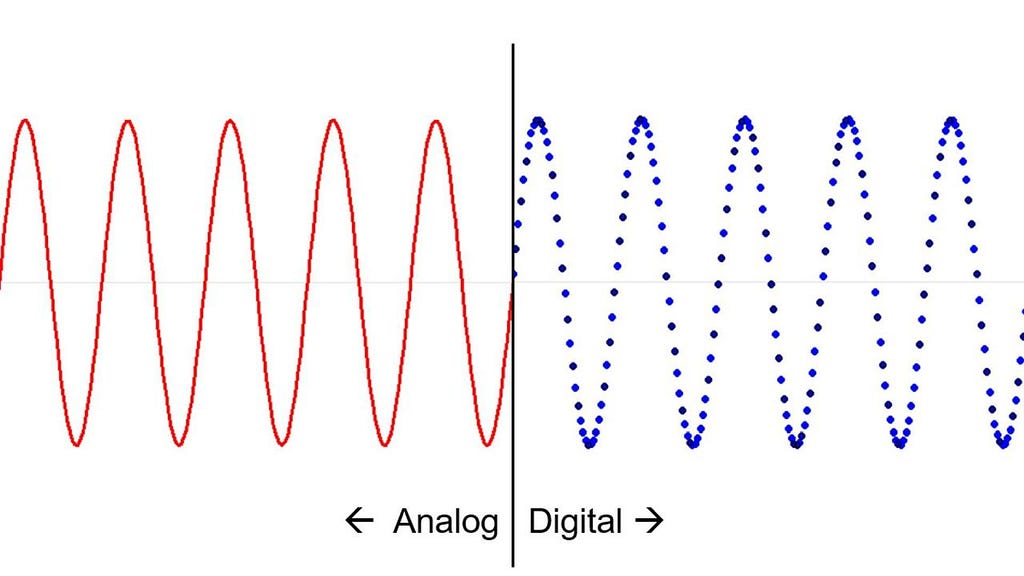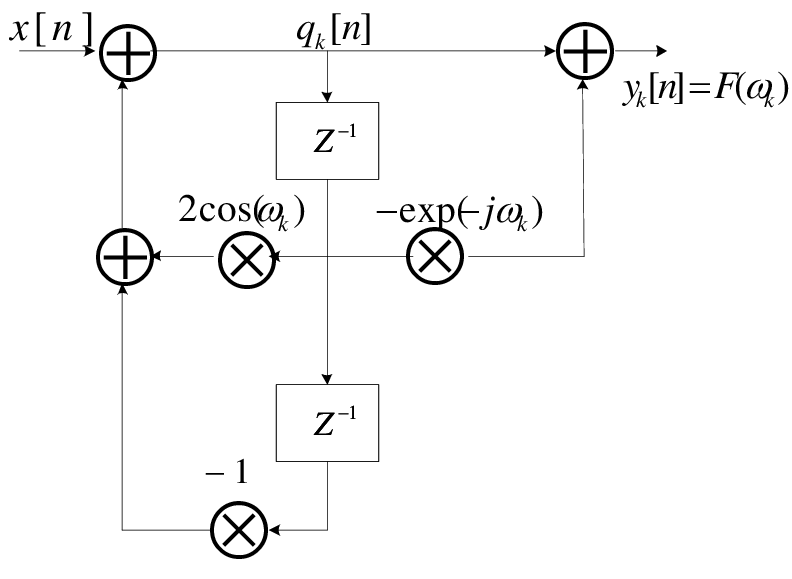# The Great Unknown — The Goertzel Algorithm

#### Beating the Fast Fourier Transform algorithm for a small number of selected frequencies is possible. Here you will learn how.

The frequency-domain analysis allows extracting information that is not obvious by simply observing a signal in time.

Nowadays frequency-domain algorithms are the backbone of many lossy compression data methods like the JPEG for image files or the MP3 for music files.

Most of these algorithms are a derivation of the Continuous Fourier Transform (CFT), originally proposed in 1822 by Jean-Baptiste Joseph Fourier, the father of modern engineering.

The problem with CFT is that it can only be applied to analogical signals, and we all live in a digital/discrete world.Differences between an analogic signal (red) and its discrete counterpart (blue).

Our computers, smartphones, satellites, and airliners are just a few examples of digital systems that work using data that have been recorded or measured in a discrete way (discrete sampling), and thus, the original CFT is not of much utility to digital devices.

The
Discrete Fourier Transform (DFT) is the natural and immediate response to the CFT in the digital world, but with some limitations related to the execution time due to the almost total absence of optimization of said algorithm.

The Fast Fourier Transform (FFT) solves this limitation by significantly improving the calculation time consumed by the discrete transform — it reduces the number of mathematical operations required to perform the same computation.

I’m sure that if you are an engineer, a mathematician, or physic you are familiar with the FFT and for sure you would have no issues applying a black box FFT algorithm to obtain the power spectral density of a discrete signal.

But what many don’t know, however, is that if you need to obtain only a few coefficients of the DFT, a much faster method is available, it’s called the Goertzel algorithm, in honor of Dr. Gerald Goertzel who first proposed the method back in 1958.

### The Goertzel Algorithm

The Goertzel algorithm can detect the components of specific frequencies in a signal, without analyzing the entire spectrum, resulting
in a shorter execution time than with the FFT.

This algorithm is very useful in the handling of DMFT (Dual-Tone Multi-Frequency) tones, more and more used nowadays in the tone recognition systems used by the companies to provide or sell services through landlines or cell phones.

It is also very useful for low-computational-power embedded systems, like those used in drone controller boards for example.

So, how does the algorithm actually works? The idea behind this is that if you manipulate the DFT formula wisely, you can end up simplifying the required number of computations to those performed in a simple second-order Infinite Impulse Response (IIR) filter.

Let’s go through it.

### The DFT as an IIR Digital Filter

In the Goertzel algorithm, a set of N samples of signal x is transformed into a set of N frequency coefficients y using the discrete Fourier transform (DFT):

`y(k) = ∑ W(nk)x(n)`

where n and k range from 0 to N-1, and the twiddle factor W(t) is defined as W(t) = exp(-j2π/Nt).

The twiddle factor has the property that W(-Nk) = 1 for all k, therefore the kᵗʰ coefficient y(k) is:

`y(k) = ∑ W(k(n-N))x(n)`

The summation can be unfolded and manipulated to make it recursive:

`y(k) = W(-k) x(N-1) + W(-2k) x(N-2) + W(-3k) x(N-3) + … + W(-k(N-1)) x(1) + W(-k N ) x(0)`
`y(k) = W(-k) [ x(N-1) + W( -k) x(N-2) + W(-2k) x(N-3) + … + W(-k(N-2)) x(1) + W(-k(N-1)) x(0) ]`
`y(k) = W(-k) [ x(N-1) + W( -k) [ x(N-2) + W( -k) x(N-3) + … + W(-k(N-3)) x(1) + W(-k(N-2)) x(0) ] ]`
`y(k) = W(-k) [ x(N-1) + W(-k) [ x(N-2) + W( -k) [ x(N-3) + … + W(-k(N-4)) x(1) + W(-k(N-3)) x(0) ] ] ]`

It is evident that the frequency coefficient y(k) can be computed in N steps using the following recursive formula:

`yn(k) = W(-k) [x(n) + yn_1(k)]`

With n ranging from 0 to N-1, andy0(k) = 0.

The recursive formula can be seen as a second-order IIR filter because it is a weighted sum of the input sample x and the previous output y.

Of course, only the final output yN_1(k) is of interest, intermediate outputs are not needed. It is possible to manipulate the coefficients of the filter so that the computation is done using real numbers, except for the last step where the final complex output yN_1(k) is computed.

The recursive formula in the z-domain is Y(z) = W(-k)[X(z) + Y(z)z^-1]. Therefore the filter transfer function is H(z) = Y(z) / X(z) = W(-k) / (1 — W(-k)z^-1). Now, multiplying the numerator and the denominator by the same quantity (1-W(+k)z^-1) we can obtain:

`H(z) = W(-k)(1-W(+k)z^-1) / [(1-W(+k)z^-1)(1-W(-k)z^-1)]`
`H(z) = [W(-k) – W(-k)W(+k) z^-1] / [1 – (W(-k)+W(+k)) z^-1 +W(-k)W(+k) z^-2)]1)(1-W(-k)z^-1)]`

If you remember, W(-k) = exp(j2π/Nk)and W(+k) = exp(-j2π/Nk), therefore W(-k)W(+k) = exp(0) = 1 and W(W(+k)+W(-k))= 2cos(2π/Nk). Thus we finally have

`H(z) = [ W(-k) –z^-1 ] / [ 1 –2cos(2pi/N k) z^-1 +z^-2 ) ]`

If the filter is implemented as Direct-II form, the status registers of the filter are real numbers, and multiplications and additions in the feedback loop are real. One complex multiplication is needed at the last step to compute the real and the imaginary part of the output y(k).Direct-II form implementation of the IIR filter of the Goertzel algorithm

Here you can find a MATLAB implementation of the Goertzel algorithm, as you can see it is really straightforward.

### When to Use the Goertzel Algorithm Instead of the FFT?

It’s only a matter of the problem’s complexity.

As a rule-of-thumb, for determining whether a radix-2 FFT or a Goertzel algorithm is more efficient, first, you have to adjust the number of terms N in the data set upward to the nearest exact power of 2. Let’s call this new parameterN_2.

The Goertzel algorithm will be the preferred solution if:

`M < 5 * N_2 * log2(N_2) / (6 * N)`

Where M is the number of frequency coefficients of the DFT to be obtained.

### Takeaways

Now that you have incorporated a new methodology to your digital signal processing toolbox, although the FFT is the most well-known method to obtain the discrete Fourier terms of a certain discrete signal, the next time you would need to compute only a bunch of them, think first if the Goertzel algorithm will provide faster computations.

Who knows, maybe the next time you are developing embedded software for a computational-power-constrained device this algorithm might come in hand. Keep it in mind!

Did you like what you have just read? Recommend this post by clicking the clap button so others can see it!

Rodney Rodríguez Robles is an aerospace engineer, cyclist, blogger, and cutting edge technology advocate, living a dream in the aerospace industry he only dreamed of as a kid. He talks about coding, the history of aeronautics, rocket science, and all the technology that is making your day by day easier.

Please check me out on the following social networks as well, I would love to hear from you! — LinkedIn, Twitter.The Great Unknown — The Goertzel Algorithm was originally published in codeburst on Medium, where people are continuing the conversation by highlighting and responding to this story.